x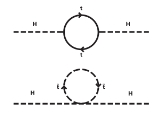Hierarchy problemEncyclopedia
In theoretical physics
Theoretical physics
Theoretical physics is a branch of physics which employs mathematical models and abstractions of physics to rationalize, explain and predict natural phenomena...

, a hierarchy problem occurs when the fundamental parameters (coupling
Coupling constant
In physics, a coupling constant, usually denoted g, is a number that determines the strength of an interaction. Usually the Lagrangian or the Hamiltonian of a system can be separated into a kinetic part and an interaction part...

s or masses) of some Lagrangian
Lagrangian mechanics
Lagrangian mechanics is a re-formulation of classical mechanics that combines conservation of momentum with conservation of energy. It was introduced by the Italian-French mathematician Joseph-Louis Lagrange in 1788....

are vastly different (usually larger) than the parameters measured by experiment. This can happen because measured parameters are related to the fundamental parameters by a prescription known as renormalization
Renormalization
In quantum field theory, the statistical mechanics of fields, and the theory of self-similar geometric structures, renormalization is any of a collection of techniques used to treat infinities arising in calculated quantities....

. Typically the renormalization parameters are closely related to the fundamental parameters, but in some cases, it appears that there has been a delicate cancellation between the fundamental quantity and the quantum corrections to it. Hierarchy problems are related to fine-tuning problem
Fine-tuning
In theoretical physics, fine-tuning refers to circumstances when the parameters of a model must be adjusted very precisely in order to agree with observations. Theories requiring fine-tuning are regarded as problematic in the absence of a known mechanism to explain why the parameters happen to...

s and problems of naturalness
Naturalness (physics)
Naturalness is the property that all parameters appearing in a theory take values of order 1...

.

Studying the renormalization in hierarchy problems is difficult, because such quantum corrections are usually power-law divergent which means that the shortest-distance physics are most important. Because we do not know the precise details of the shortest-distance theory of physics (quantum gravity
Quantum gravity
Quantum gravity is the field of theoretical physics which attempts to develop scientific models that unify quantum mechanics with general relativity...

), we cannot even address how this delicate cancellation between two large terms occurs. Therefore, researchers postulate new physical phenomena that resolve hierarchy problems without fine tuning.

## The Higgs Mass

In particle physics
Particle physics
Particle physics is a branch of physics that studies the existence and interactions of particles that are the constituents of what is usually referred to as matter or radiation. In current understanding, particles are excitations of quantum fields and interact following their dynamics...

, the most important hierarchy problem is the question that asks why the weak force is 1032 times stronger than gravity. Both of these forces involve constants of nature, Fermi's constant for the weak force and Newton's constant for gravity. Furthermore if the Standard Model
Standard Model
The Standard Model of particle physics is a theory concerning the electromagnetic, weak, and strong nuclear interactions, which mediate the dynamics of the known subatomic particles. Developed throughout the mid to late 20th century, the current formulation was finalized in the mid 1970s upon...

is used to calculate the quantum corrections to Fermi's constant, it appears that Fermi's constant is unnaturally large and should be closer to Newton's constant, unless there is a delicate cancellation between the bare value of Fermi's constant and the quantum corrections to it.More technically, the question is why the Higgs boson
Higgs boson
The Higgs boson is a hypothetical massive elementary particle that is predicted to exist by the Standard Model of particle physics. Its existence is postulated as a means of resolving inconsistencies in the Standard Model...

is so much lighter than the Planck mass (or the grand unification energy, or a heavy neutrino mass scale): one would expect that the large quantum contributions to the square of the Higgs boson mass would inevitably make the mass huge, comparable to the scale at which new physics appears, unless there is an incredible fine-tuning
Fine-tuning
In theoretical physics, fine-tuning refers to circumstances when the parameters of a model must be adjusted very precisely in order to agree with observations. Theories requiring fine-tuning are regarded as problematic in the absence of a known mechanism to explain why the parameters happen to...

It should be remarked that the problem cannot even be formulated in the strict context of the Standard Model, for the Higgs mass cannot be calculated. In a sense, the problem amounts to the worry that a future theory of fundamental particles, in which the Higgs boson mass will be calculable, should not have excessive fine-tunings. Implicit in the reasoning that leads to the fine-tuning concern is the unsubstantiated assumption that little physics other than renormalization group scaling exists between the Higgs scale and the grand unification energy. As these two scales are separated by at least 11 orders of magnitude, this "big desert
Desert (particle physics)
In particle physics, the desert refers to a theorized gap in energy scales between the TeV scale and the GUT scale in which no new physics appears. The idea of the desert was motivated by the observation of approximate, order of magnitude, gauge coupling unification at the GUT scale...

" assumption is seen as unlikely to be true by most physicists outside the string discipline.

If one accepts the big-desert assumption and thus the existence of a Hierarchy Problem, some new mechanism at Higgs scale becomes necessary to avoid the fine-tuning.

The most popular theory—but not the only proposed theory—to solve the hierarchy problem is supersymmetry
Supersymmetry
In particle physics, supersymmetry is a symmetry that relates elementary particles of one spin to other particles that differ by half a unit of spin and are known as superpartners...

. This explains how a tiny Higgs mass can be protected from quantum corrections. Supersymmetry removes the power-law divergences of the radiative corrections to the Higgs mass; however, there is no understanding of why the Higgs mass is so small in the first place which is known as the mu problem
Mu problem
In theoretical physics, the μ problem is a problem of supersymmetric theories, concerned with understanding the parameters of the theory.The supersymmetric Higgs mass parameter μ appears as the following term in the superpotential: μHuHd. It is necessary to provide a mass for the fermionic...

. Furthermore, there is no natural way to break supersymmetry so far below the grand unification energy, so what one gets is basically substituting the original Hierarchy Problem of Higgs with a new Hierarchy Problem of supersymmetry breaking.

## Supersymmetric Solution

Each particle that couples to the Higgs field has a Yukawa coupling λf. The coupling with the Higgs field for fermions gives an interaction term LYukawa=-λf ψ ̅Hψ, ψ being the Dirac Field and H the Higgs Field. Also, the mass of a fermion is proportional to its Yukawa coupling, meaning that the Higgs boson will couple most to the most massive particle. This means that the most significant corrections to the Higgs mass will originate from the heaviest particles, most prominently the top quark. By applying the Feynman rules, one gets the quantum corrections to the Higgs mass squared from a fermion to be: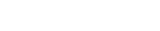The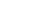is called the ultraviolet cutoff and is the scale up to which the Standard Model is valid. If we take this scale to be the Planck scale, then we have the quadratically diverging Lagrangian. However, suppose there existed two complex scalars (taken to be spin - 0) such that:

λS= |λf|2 (the couplings to the Higgs are exactly are the same).

Then by the Feynman rules, the correction (from both scalars) is: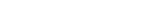(Note that the contribution here is positive. This is because of the spin-statistics theorem, which means that fermions will have a negative contribution and bosons a positive contribution. This fact is exploited)
This gives a total contribution to the Higgs mass to be zero if we include both the fermionic and bosonic particles. Supersymmetry
Supersymmetry
In particle physics, supersymmetry is a symmetry that relates elementary particles of one spin to other particles that differ by half a unit of spin and are known as superpartners...

is an extension of this that creates 'superpartners' for all Standard Model particles.

This section adapted from Stephen P. Martin's "A Supersymmetry Primer" on arXiv.

## Solution via Extra Dimensions

If we live in a 3+1 dimensional world, then we calculate the Gravitational Force via Gauss' law for gravity
Gauss' law for gravity
In physics, Gauss's law for gravity, also known as Gauss's flux theorem for gravity, is a law of physics which is essentially equivalent to Newton's law of universal gravitation...

: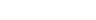(1)
which is simply the Newton's law of gravitation. Note that Newton's constant G can be rewritten in terms of the Planck Mass.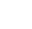If we extend this idea to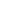extra dimensions, then we get: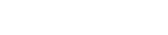(2)

where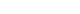is the 3+1+dimensional Planck mass. However, we are assuming that these extra dimensions are the same size as the normal 3+1 dimensions. Let us say that the extra dimensions are of size n <<< than normal dimensions. If we let r << n, then we get (2). However, if we let r >> n, then we get our usual Newton's law. However, when r >> n, the flux in the extra dimensions becomes a constant, because there is no extra room for gravitational flux to flow through. Thus the flux will be proportional to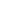because this is the flux in the extra dimensions. The formula is: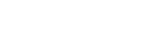which gives: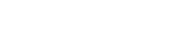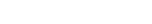Thus the fundamental Planck Mass (the extra dimensional one) could actually be small, meaning that gravity is actually strong, but this must be compensated by the number of the extra dimensions and their size. Physically, this means that gravity is weak because there is a loss of flux to the extra dimensions.

This section adapted from "Quantum Field Theory in a Nutshell" by A. Zee.

### Braneworld models

In 1998 Nima Arkani-Hamed
Nima Arkani-Hamed
Nima Arkani-Hamed is a leading Canadian American theoretical physicist with interests in high-energy physics, string theory and cosmology....

, Savas Dimopoulos
Savas Dimopoulos
Savas Dimopoulos is a Greek particle physicist at Stanford University. He was born in Istanbul, Turkey and later moved to Athens due to ethnic tensions in Turkey during the 1950s and 1960s. Dimopoulos studied as an undergraduate at the University of Houston...

, and Gia Dvali
Gia Dvali
Georgi Dvali is a professor of physics at LMU Munich and a director at the Max Planck Institute for Physics. He received his Ph.D. in high energy physics and cosmology from Tbilisi State University, Georgia in 1992...

proposed the ADD model, also known as the model with large extra dimensions, is an alternative scenario to explain the weakness of gravity relative to the other forces. This theory requires that the fields of the Standard Model
Standard Model
The Standard Model of particle physics is a theory concerning the electromagnetic, weak, and strong nuclear interactions, which mediate the dynamics of the known subatomic particles. Developed throughout the mid to late 20th century, the current formulation was finalized in the mid 1970s upon...

are confined to a four-dimensional membrane
Membrane (M-Theory)
In theoretical physics, a membrane, brane, or p-brane is a spatially extended mathematical concept that appears in string theory and related theories...

, while gravity propagates in several additional spatial dimensions that are large compared to the Planck scale
Planck scale
In particle physics and physical cosmology, the Planck scale is an energy scale around 1.22 × 1019 GeV at which quantum effects of gravity become strong...

.

In 1998/99 Merab Gogberashvili published on Arxiv
ArXiv
The arXiv |Chi]], χ) is an archive for electronic preprints of scientific papers in the fields of mathematics, physics, astronomy, computer science, quantitative biology, statistics, and quantitative finance which can be accessed online. In many fields of mathematics and physics, almost all...

(and subsequently in peer-reviewed journals) a number of articles where showed that if the Universe is considered as a thin shell (a mathematical synonym
Synonym
Synonyms are different words with almost identical or similar meanings. Words that are synonyms are said to be synonymous, and the state of being a synonym is called synonymy. The word comes from Ancient Greek syn and onoma . The words car and automobile are synonyms...

for "brane") expanding in 5-dimensional space then there is a possibility to obtain one scale for particle theory corresponding to the 5-dimensional cosmological constant
Cosmological constant
In physical cosmology, the cosmological constant was proposed by Albert Einstein as a modification of his original theory of general relativity to achieve a stationary universe...

and Universe thickness, and thus to solve the hierarchy problem. It was also shown that four-dimensionality of the Universe is the result of stability
Stability theory
In mathematics, stability theory addresses the stability of solutions of differential equations and of trajectories of dynamical systems under small perturbations of initial conditions...

requirement since the extra component of the Einstein equations giving the localized solution for matter
Matter
Matter is a general term for the substance of which all physical objects consist. Typically, matter includes atoms and other particles which have mass. A common way of defining matter is as anything that has mass and occupies volume...

fields coincides with the one of the conditions of stability.

Subsequently, there were proposed the closely related Randall-Sundrum
Randall-Sundrum model
In physics, Randall–Sundrum models imagine that the real world is a higher-dimensional Universe described by warped geometry...

scenarios which offered their solution to the hierarchy problem.

### Empirical tests

Until now, no experimental or observational evidence of extra dimensions
Extra dimensions
Several speculative physical theories have introduced extra dimensions of space for various reasons:*Kaluza-Klein theory introduces extra dimensions to explain the fundamental forces other than gravity ....

has been officially reported. An analysis of results from the Large Hadron Collider
The Large Hadron Collider is the world's largest and highest-energy particle accelerator. It is expected to address some of the most fundamental questions of physics, advancing the understanding of the deepest laws of nature....

in December 2010 severely constrains theories with large extra dimensions.

## The Cosmological Constant

In physical cosmology
Physical cosmology
Physical cosmology, as a branch of astronomy, is the study of the largest-scale structures and dynamics of the universe and is concerned with fundamental questions about its formation and evolution. For most of human history, it was a branch of metaphysics and religion...

, current observations in favor of an accelerating universe
Accelerating universe
The accelerating universe is the observation that the universe appears to be expanding at an increasing rate, which in formal terms means that the cosmic scale factor a has a positive second derivative, implying that the velocity at which a given galaxy is receding from us should be continually...

imply the existence of a tiny, but nonzero cosmological constant
Cosmological constant
In physical cosmology, the cosmological constant was proposed by Albert Einstein as a modification of his original theory of general relativity to achieve a stationary universe...

. This is a hierarchy problem very similar to that of the Higgs boson mass problem, since the cosmological constant is also very sensitive to quantum corrections. It is complicated, however, by the necessary involvement of General Relativity
General relativity
General relativity or the general theory of relativity is the geometric theory of gravitation published by Albert Einstein in 1916. It is the current description of gravitation in modern physics...

in the problem and may be a clue that we do not understand gravity on long distance scales (such as the size of the universe
Universe
The Universe is commonly defined as the totality of everything that exists, including all matter and energy, the planets, stars, galaxies, and the contents of intergalactic space. Definitions and usage vary and similar terms include the cosmos, the world and nature...

today). While quintessence
Quintessence (physics)
In physics, quintessence is a hypothetical form of dark energy postulated as an explanation of observations of an accelerating universe. It has been proposed by some physicists to be a fifth fundamental force...

has been proposed as an explanation of the acceleration of the Universe, it does not actually address the cosmological constant hierarchy problem in the technical sense of addressing the large quantum corrections. Supersymmetry does not permit to address the cosmological constant problem, since supersymmetry cancels the M4Planck contribution but not the M2Planck one (quadratically diverging).